# Use and validity of principles of extremum of entropy production in the study of complex systems

A. Heitor Reis

University of Évora, Department of Physics and Geophysics Center of Évora, Colégio Luis Verney, R. Romão Ramalho, 59, 7002-554, Évora, Portugal

Tel.: +351 967324948; fax: +351 266 745394.

http://dx.doi.org/10.1016/j.aop.2014.03.013

Annals of Physics 346 (2014) 22–27

## Highlights

• The principles of extremum of entropy production are not first principles.
• They result from the maximization of conductivities under appropriate constraints.
• The conditions of their validity are set explicitly.
• Some long-standing controversies are discussed and clarified.

## Article Info

Article history:
Accepted 29 March 2014
Available online 4 April 2014

Keywords:
Entropy production
Extremum principles
Non-equilibrium

## Abstract

It is shown how both the principles of extremum of entropy production, which are often used in the study of complex systems, follow from the maximization of overall system conductivities, under appropriate constraints. In this way, the maximum rate of entropy production (MEP) occurs when all the forces in the system are kept constant. On the other hand, the minimum rate of entropy production (mEP) occurs when all the currents that cross the system are kept constant. A brief discussion on the validity of the application of the mEP and MEP principles in several cases, and in particular to the Earth’s climate is also presented.

## 1. Introduction

In the celebrated textbook ‘‘The Feynman Lectures on Physics’’  the authors stated that: ‘‘minimum principles sprang in one way or another from the least action principle of mechanics and electrodynamics. But there is also a class that does not. As an example, if currents are made to go through a piece of material obeying Ohm’s Law, the currents distribute themselves inside the piece so that the rate at which heat is generated is as little as possible. Also, we can say – if things are kept isothermal – that the rate at which heat is generated is as little as possible’’.

The above statement marks just one moment in the long-standing debate about the legitimacy of using principles of extremum of entropy production rate as a basis to explain the behavior of certain systems out of equilibrium.

The principle of Minimum Entropy Production rate (mEP) was first proposed by Prigogine [2,3] as a rule governing open systems at nonequilibrium stationary states. The justification of mEP presented by Prigogine still continues to be the subject of heated controversy (see Refs. [4,5]).

Maximum Entropy Production rate principle (MEP) was proposed in 1956 by Ziman  in the form: ‘‘Consider all distributions of currents such that the intrinsic entropy production equals the extrinsic entropy production for the given set of forces. Then, of all current distributions satisfying this condition, the steady state distribution makes the entropy production a maximum’’. A practical application was put forward by Paltridge [7–9] who proposed that the Earth’s climate structure could be explained through the MEP principle. As in the case of the mEP principle, comments have appeared in the literature suggesting that the MEP principle as a basis for understanding the Earth-Atmosphere system is far from simple and universal (see Refs. [10,11]).

Recent work on mEP and MEP principles has focused either on the conceptual development and foundations of both principles [12–16], or on their applications to various systems, and namely the Earth’s climate [17–19].

## 2. Extrema of entropy production

In this paper we show that the so-called mEP and MEP principles may be derived (each one under two different sets of constraints) from the maximization of the conductivities that couple flows with the forces that drive such flows. The maximization of the conductivities follows from the general principle of maximization of ‘‘global flow access’’, known as the Constructal Law, which was first put forward in 1997 by Bejan  in the form: ‘‘For a finite-size system to persist in time (to live), it must evolve in such a way that it provides easier access to the imposed (global) currents that flow through it’’. The Constructal Law entails generation of flow configuration in such a way that it provides the highest global conductivity compatible with the existing constraints, and has successfully explained shapes and patterns of the animate [21,22] and inanimate [23–25] systems (see also some Constructal Law reviews [26–28]). In the general case of N forces Fi and N flows Ji (forces and flows are represented here as vectors), the entropy production rate σ is given by :where the symbol ◦ means dot product, i, j = 1, . . . , N, and Fk = ∇Φk, i.e. the gradients of the potentials Φ = {T , P, µ, . . . , φ}, are the forces that drive the flows Ji, which in the linear regime is read :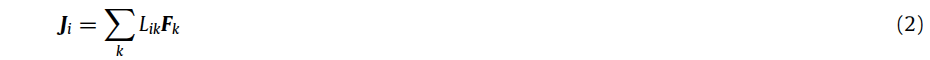where Lik is the phenomenological coefficient that couples force Fk with flow Ji. In Eqs. (1) and (2) as well in the following we consider that the indices i and j run from 1 to N. With the help of Eq. (2), the entropy production rate – Eq. (1) – may be rewritten as: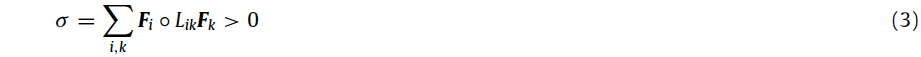which, according to the Second Law is always positive or null. Here, because we are dealing with non-equilibrium systems we consider σ > 0. Moreover, from Eq. (3) one concludes that the matrix [L] is symmetric, i.e., Lik = Lki, and positive definite .

### 2.1. Fixed forces

First, let us consider the case when all the forces Fk are kept constant, and then take the differential of both members of Eq. (3):which, according to the Constructal Law must be null. In fact, ‘‘easiest flow access’’ occurs when all Lik conductivities are at their maxima, i.e. when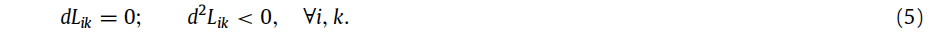Hence, for the second order differential of σ one must have:Therefore, from Eqs. (4) and (6) one can draw the following conclusion: ‘‘In the case when all the forces driving the flows are kept constant the entropy production rate reaches the maximum value that is compatible with the existing constraints’’.

Moreover, from Eq. (6) one also concludes that the matrix [−d2L] is positive definite.

### 2.2. Fixed flows

Now, let us consider the case when all the flows Ji are kept constant, together with Eq. (3). Because the matrix [L] is symmetric, i.e., Lik = Lki, and positive definite, has an inverse matrix [Λ] = [L]−1 that is also positive definite . Therefore, from Eq. (2) we can write: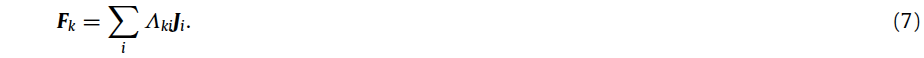Hence, in this case, Eq. (1) reads: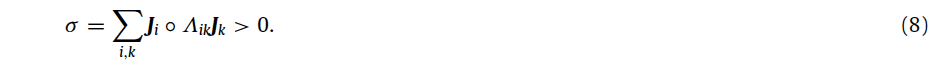By taking the differential of both sides of Eq. (8), and noting that:and invoking the Constructal Law (Eqs. (5)) again, one obtains: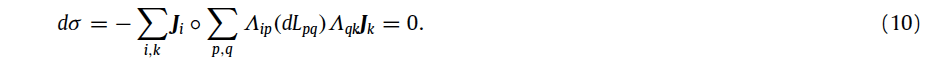Now, by taking the second order differential of both sides of Eq. (10), and using Eq. (9) to notice that dLik = 0 ⇒ ik = 0, and considering Eqs. (5) one has:In Eq. (11) we have used the property that the product of two positive definite matrices, [Λ] and [−d2L] is also positive definite .

Therefore, Eqs. (10) and (11) prove that ‘‘In the case when all the flows are kept constant, the entropy production rate reaches the minimum value that is compatible with the existing constraints’’.

In the more general case when Fk, k = l, . . . , m, and Ji, i = n, . . . , r are kept fixed, and taking into account Eqs. (1)–(5) and (7)–(10), the entropy production rate reaches an extremum if any of the following conditions is met:Hence, the more the first members of Eqs. (12) deviate from zero, the less the system shall be in line with any of the principles of extremum of the entropy production rate. This could help explain the problems with the use of both the mEP and MEP principles in some complex cases (see Refs. [4,5,10,11]). However, in the cases when Eqs. (5) are met, then by using equations analogous to Eqs. (6) and (11) one can verify if systems are governed either by mEP or MEP principles.

## 3. MEP AND mEP principles as corollaries of the Constructal Law

The extrema of entropy production rate, which are often presented as principles governing the behavior of certain systems, are in fact corollaries of the Constructal Law (Eq. (5)) in certain specific situations.

Therefore, if all the forces are kept fixed, the Constructal Law (maximal flow access, or equivalently, maximal global conductivity arrangement) entails maximal rate of entropy production (MEP). This is for instance the case of the Sun–Earth system. The blackbody temperature at which the Sun emits the energy that reaches the Earth is constant (TS = 5778 K) while the blackbody temperature of the Earth, TE is about 254.4 K and constant at the climatic scale . Hence, according to the Constructal Law, because the Earth system ‘‘processes’’ the energy coming from the Sun at a fixed ‘‘force’’, which is proportional to (TS − TE )(TS TE )−1, the global Earth system must operate under the rule of maximum entropy production rate (MEP). This is a general rule for the global system, and namely shall hold at least approximately in the Climate system, therefore clearing up the relative success of Paltridge’s explanation [7–9] of the global state of the Earth’s climate by MEP principle. As regards subsystems of the climatic system only approximately we can consider that some of the mesoscale forces are kept constant, instead multiple forces and flows are not constant. This poses the problem of verifying the conditions of applicability of MEP principle at the pertinent scale in terms of Eqs. (12), and might help explain why MEP appears not to hold for certain subsystems .

Despite Constructal Law requires maximum rate of global entropy production in the Earth, it does not forbid that this objective is reached through the global arrangement of entropy production that includes processes in which entropy production rate is at its minimum, together with other processes in which it is at its maximum. For example, in the cases in which the flow rate is fixed, as it happens when water inflow through precipitation over a territory balances water outflow carried by the streams, at least approximately, it is expected that the flow organizes itself in such a way that the overall conductivity arrangement leads to a minimum of the entropy production rate . In this case, Bejan  showed that the flow organization leads to a minimum of the driving force (water pressure head over the territory). Because the flow rate is fixed, minimal pressure head entails minimal entropy production rate, therefore confirming the general rule for this case.

At a larger scale one finds another process in which entropy generation rate is at its maximum: that of transport of the excess heat from the Earth’s equator (at temperature TH ) to the poles which are at a lower temperature TL. In this case, the global flow organizes itself in patterns that enable maximal ‘‘global heat conductivity’’ , and because heat flows under a fixed force proportional to (TH − TL)(TH TL)−1, maximal entropy production rate is expected to occur.

It is also interesting to analyze here the ‘‘Theorem of Minimum Entropy Production’’ in Prigogine’s formulation : ‘‘In the linear regime, the total entropy production in a system subject to flow of energy and matter, reaches a minimum value at the nonequilibrium stationary state’’. In the proof offered for the case of two forces and two flows, though Prigogine fixes one of the forces, he really fixes both flows thus reaching the conclusion that entropy production rate is at its minimum. As we have shown before, for the case in which all flows are fixed, the same result of minimum entropy production rate is anticipated for the much general case of any number of forces and flows.

The problem that puzzled Feynman : ‘‘. . . if currents are made to go through a piece of material obeying Ohm’s Law, the currents distribute themselves inside the piece so that the rate at which heat is generated is as little as possible. Also, we can say – if things are kept isothermal – that the rate at which heat is generated is as little as possible’’, finds a straightforward explanation in the context developed here. In fact, Feynman fixes the ‘‘currents (that) are made to go through a piece of material’’ therefore it follows that in such conditions, because the entropy production rate is at its minimum, hence as a result ‘‘the rate at which heat is generated is as little as possible’’.

Surely it is not easy to find ‘‘pure’’ cases in which all forces, or all flows are fixed, instead mixed conditions are more likely to occur. Mixed combinations in which only a part of the forces and flows are fixed are also possible to be considered in terms of Eqs. (12). However, these cases require a more complex treatment, for the reason that the respective entropy production regimes do not appear so clearly as in the extreme cases in which either all forces are fixed, or that all flows are fixed.

## 4. Conclusions

We have shown that either the principle of minimum entropy production rate (mEP), or that of maximum entropy production rate (MEP), can be derived by maximizing the overall conductivity associated with each flow that crosses the system, under specific constraints. Thus, the entropy production rate is minimal when all the flows are kept constant. Likewise, the entropy production rate is maximal when all the forces that generate the flows are kept constant.

The principle of ‘‘maximum flow access’’, known as the Constructal Law appears as the theoretical foundation behind the mEP and MEP principles. It becomes clear that the Constructal Law is distinct from the Second Law, and is a fundamental law that rules the generation of configuration and structure in systems out of equilibrium.

## Acknowledgments

The author acknowledges the funding provided by the Évora Geophysics Centre, Portugal, under the contract with Fundação para a Ciência e a Tecnologia (the Portuguese Science and Technology Foundation), Pest/OE/CTE/UI0078/2014.

This site uses Akismet to reduce spam. Learn how your comment data is processed.